U.S. Department of Transportation
1200 New Jersey Avenue, SE
Washington, DC 20590
202-366-4000

Federal Highway Administration Research and Technology
Coordinating, Developing, and Delivering Highway Transportation Innovations

 REPORT This report is an archived publication and may contain dated technical, contact, and link information
 Federal Highway Administration > Publications > Research Publications > 13085 > Chapter 2. Theoretical Background for Nonlinear ACoustics
 Publication Number:  FHWA-HRT-13-085    Date:  October 2013
 Publication Number: FHWA-HRT-13-085 Date: October 2013

# Accelerated Determination of ASR Susceptibility During Concrete Prism Testing Through Nonlinear Resonance Acoustic Spectroscopy

## CHAPTER 2. Theoretical background For nonlinear acoustics

It is well known that cracks within a material decrease its resonance frequency by decreasing the overall stiffness of the structure. In addition to this linear change in frequency, researchers have demonstrated that cracks are also responsible for nonlinear effects, including the strain amplitude dependent resonance frequency shift.(16,17) Microcracks inside a material form a network that acts as a nonlinear bond system. The nonlinear behavior of this bond system can be attributed to Hertzian contact of crack faces and/or opening and closing of cracks in response to wave motion. Using the phenomenological model for hysteresis and classical nonlinear constitutive relationships, researchers have shown that the nonlinear stress–strain relationship can be shown as the equation in figure 1.(9,13).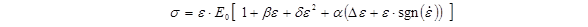Figure 1. Equation. Nonlinear stress–strain relationship

where
σ  =  stress,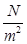Ε0 =  linear elastic modulus,β  =  coefficient of quadratic anharmonicity
δ   =  coefficient of cubic anharmonicity
ε =  strain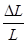, where L is length
α  =  measure of the material hysteresis
Δε   =  strain amplitude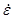=  strain rate,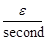sgn()=1  if> 0 , -1 if< 0 , and 0 if= 0

Assuming that effects of hysteresis are dominant in microcracked materials, the relationship between frequency shift and strain amplitude shown in figure 2 is valid for low levels of strain excitation.(13)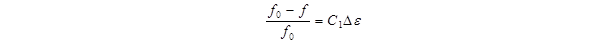Figure 2. Equation. Relationship between frequency shift and strain amplitude

where
f0  =  linear resonance frequency, Hz
f  =  resonance frequency at increased excitation amplitude, Hz
C1  =  coefficient proportional to material hysteresis

At higher amplitudes, there will also be an additional quadratic term for the strain amplitude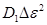; however, because the experiments are performed at low levels of strain excitation, this higher-order term can be ignored. In these experiments, the amplitude of the signal, A, which is proportional to the strain amplitude, Δε, is measured instead of the strain amplitude. As a result, the absolute hysteresis parameter, α, is not measured. Instead, a scaled hysteresis parameter (η) proportional to α is used as a measure of the material's nonlinearity. The relationship used in this investigation is given by the equation shown in figure 3.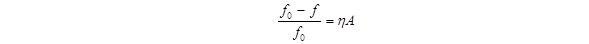Figure 3. Equation. Relationship between the frequency shift, the scaled hysteresis parameter, and the signal amplitude

Chapter 4 explains in detail the extraction of the parameter η  from recorded data. An additional effect observed for hysteretic materials is the increase in damping for the sample. The equation in figure 4 shows that a linear relationship exists between the change in damping and the strain amplitude.(13)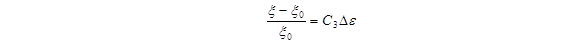Figure 4. Equation. Relationship between change in damping and strain amplitude

where
ξ0  =  linear damping rate
ξ  =  damping rate at increased excitation amplitude
C3  =  coefficient proportional to material hysteresis

Because the signal amplitude is proportional to strain amplitude, the relationship between the change in damping and the signal amplitude is the equation shown in figure 5: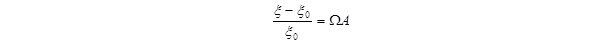Figure 5. Equation. Relationship between the change in damping and the signal amplitude

where Ω  is termed the nonlinear damping parameter.

Because the nonlinearity is attributed to nonlinear interaction of cracks, relatively large and open cracks will not contribute to nonlinearity. Under this assumption, the nonlinearity parameter can be thought of as an “instantaneous” measure of nonlinearity. Because the measurements for tracking nonlinearity in CPT samples are taken at rather long intervals of time, the “cumulative” nonlinearity (ηc) can be measured by integration, as shown in the equation in figure 6.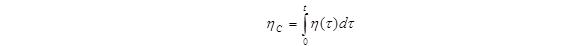Figure 6. Equation. Integral to calculate “cumulative” nonlinearity

With experimental data, a Riemann sum can be used to approximate this integral (see figure 7).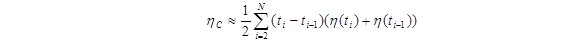Figure 7. Equation. Approximation of integral to calculate”cumulative” nonlinearity using a Riemann sum